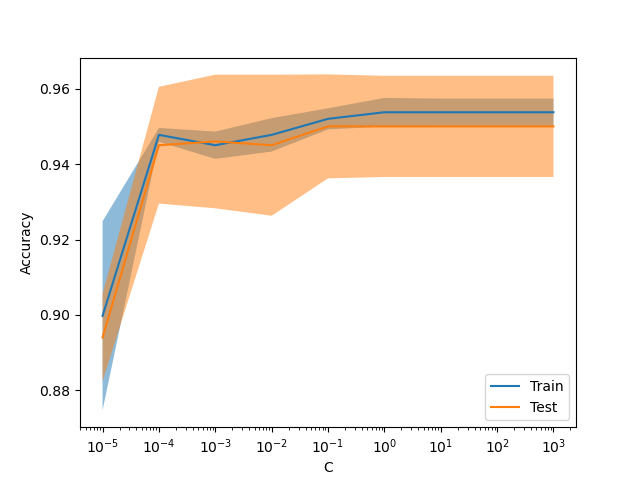# Release Highlights for scikit-learn 1.3¶

We are pleased to announce the release of scikit-learn 1.3! Many bug fixes and improvements were added, as well as some new key features. We detail below a few of the major features of this release. For an exhaustive list of all the changes, please refer to the release notes.

pip install --upgrade scikit-learn


or with conda:

conda install -c conda-forge scikit-learn


We are in the process of introducing a new way to route metadata such as sample_weight throughout the codebase, which would affect how meta-estimators such as pipeline.Pipeline and model_selection.GridSearchCV route metadata. While the infrastructure for this feature is already included in this release, the work is ongoing and not all meta-estimators support this new feature. You can read more about this feature in the Metadata Routing User Guide. Note that this feature is still under development and not implemented for most meta-estimators.

Third party developers can already start incorporating this into their meta-estimators. For more details, see metadata routing developer guide.

## HDBSCAN: hierarchical density-based clustering¶

Originally hosted in the scikit-learn-contrib repository, cluster.HDBSCAN has been adpoted into scikit-learn. It’s missing a few features from the original implementation which will be added in future releases. By performing a modified version of cluster.DBSCAN over multiple epsilon values simultaneously, cluster.HDBSCAN finds clusters of varying densities making it more robust to parameter selection than cluster.DBSCAN. More details in the User Guide.

import numpy as np
from sklearn.cluster import HDBSCAN
from sklearn.metrics import v_measure_score

print(f"number of digits: {len(np.unique(true_labels))}")

hdbscan = HDBSCAN(min_cluster_size=15).fit(X)
non_noisy_labels = hdbscan.labels_[hdbscan.labels_ != -1]
print(f"number of clusters found: {len(np.unique(non_noisy_labels))}")

print(v_measure_score(true_labels[hdbscan.labels_ != -1], non_noisy_labels))

number of digits: 10
number of clusters found: 11
0.9694149248180188


## TargetEncoder: a new category encoding strategy¶

Well suited for categorical features with high cardinality, preprocessing.TargetEncoder encodes the categories based on a shrunk estimate of the average target values for observations belonging to that category. More details in the User Guide.

import numpy as np
from sklearn.preprocessing import TargetEncoder

X = np.array([["cat"] * 30 + ["dog"] * 20 + ["snake"] * 38], dtype=object).T
y = [90.3] * 30 + [20.4] * 20 + [21.2] * 38

enc = TargetEncoder(random_state=0)
X_trans = enc.fit_transform(X, y)

enc.encodings_

[array([90.3, 20.4, 21.2])]


## Missing values support in decision trees¶

The classes tree.DecisionTreeClassifier and tree.DecisionTreeRegressor now support missing values. For each potential threshold on the non-missing data, the splitter will evaluate the split with all the missing values going to the left node or the right node. More details in the User Guide.

import numpy as np
from sklearn.tree import DecisionTreeClassifier

X = np.array([0, 1, 6, np.nan]).reshape(-1, 1)
y = [0, 0, 1, 1]

tree = DecisionTreeClassifier(random_state=0).fit(X, y)
tree.predict(X)

array([0, 0, 1, 1])


## New display model_selection.ValidationCurveDisplay¶

model_selection.ValidationCurveDisplay is now available to plot results from model_selection.validation_curve.

from sklearn.datasets import make_classification
from sklearn.linear_model import LogisticRegression
from sklearn.model_selection import ValidationCurveDisplay

X, y = make_classification(1000, 10, random_state=0)

_ = ValidationCurveDisplay.from_estimator(
LogisticRegression(),
X,
y,
param_name="C",
param_range=np.geomspace(1e-5, 1e3, num=9),
score_type="both",
score_name="Accuracy",
)## Gamma loss for gradient boosting¶

The class ensemble.HistGradientBoostingRegressor supports the Gamma deviance loss function via loss="gamma". This loss function is useful for modeling strictly positive targets with a right-skewed distribution.

import numpy as np
from sklearn.model_selection import cross_val_score
from sklearn.datasets import make_low_rank_matrix

n_samples, n_features = 500, 10
rng = np.random.RandomState(0)
X = make_low_rank_matrix(n_samples, n_features, random_state=rng)
coef = rng.uniform(low=-10, high=20, size=n_features)
y = rng.gamma(shape=2, scale=np.exp(X @ coef) / 2)
cross_val_score(gbdt, X, y).mean()

0.46858513287221654


## Grouping infrequent categories in preprocessing.OrdinalEncoder¶

Similarly to preprocessing.OneHotEncoder, the class preprocessing.OrdinalEncoder now supports aggregating infrequent categories into a single output for each feature. The parameters to enable the gathering of infrequent categories are min_frequency and max_categories. See the User Guide for more details.

from sklearn.preprocessing import OrdinalEncoder
import numpy as np

X = np.array(
[["dog"] * 5 + ["cat"] * 20 + ["rabbit"] * 10 + ["snake"] * 3], dtype=object
).T
enc = OrdinalEncoder(min_frequency=6).fit(X)
enc.infrequent_categories_

[array(['dog', 'snake'], dtype=object)]


Total running time of the script: (0 minutes 1.731 seconds)

Gallery generated by Sphinx-Gallery